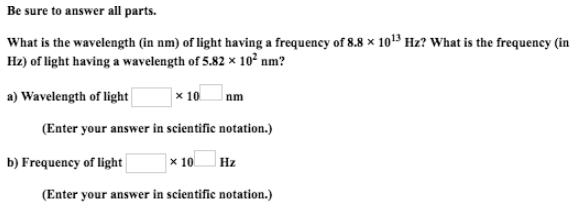# Problem: What is the wavelength (in nm) of light having a frequency of 8.8 x 10 13 Hz? What is the frequency (in Hz) of light having a wavelength of 5.82 x 102 nm?

###### FREE Expert Solution###### Problem Details

What is the wavelength (in nm) of light having a frequency of 8.8 x 10 13 Hz? What is the frequency (in Hz) of light having a wavelength of 5.82 x 102 nm?